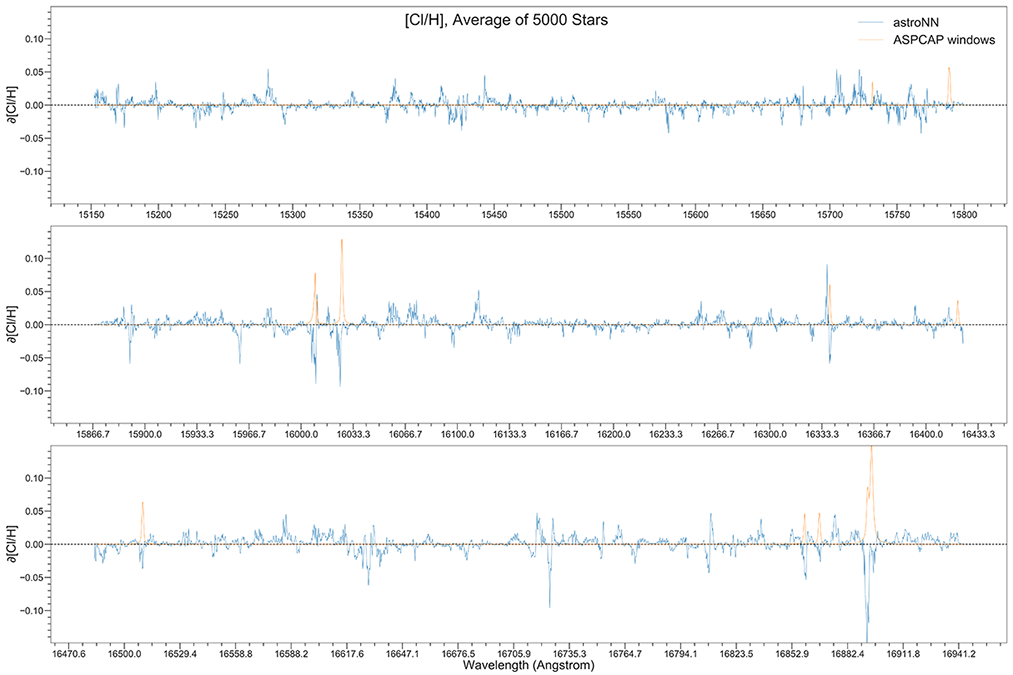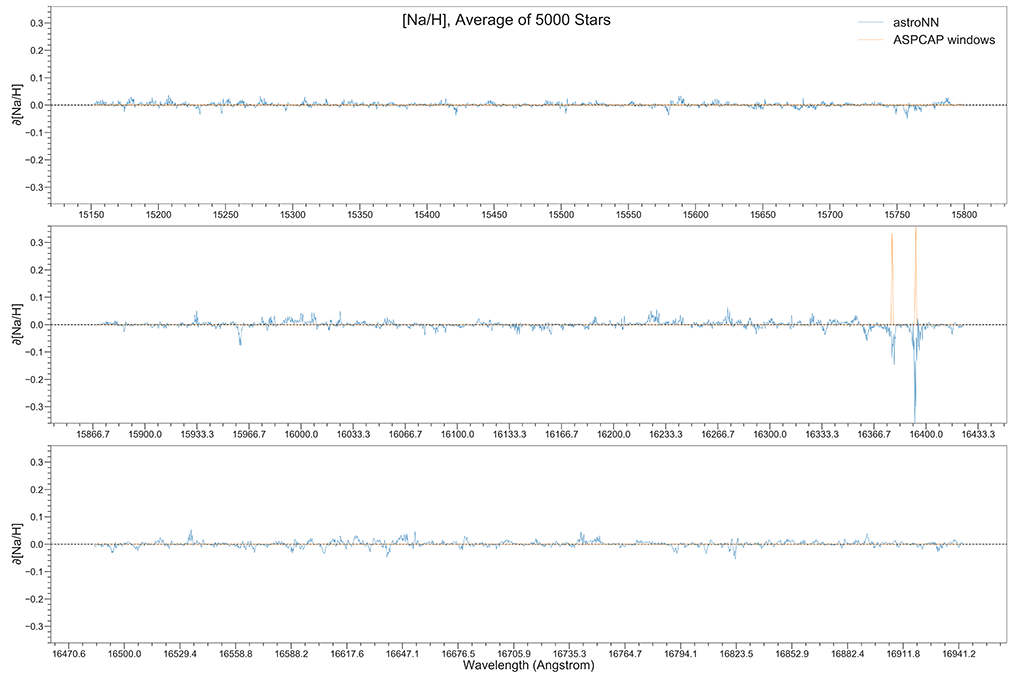# APOGEE Spectra with Bayesian Neural Net - astroNN.models.ApogeeBCNN¶

class astroNN.models.ApogeeBCNN.ApogeeBCNN(lr=0.0005, dropout_rate=0.3)

Class for Bayesian convolutional neural network for stellar spectra analysis

History: 2017-Dec-21 - Written - Henry Leung (University of Toronto)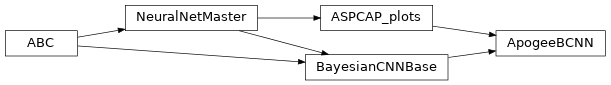Although in theory you can feed any 1D data to astroNN neural networks. This tutorial will only focus on spectra analysis.

from astroNN.models import ApogeeBCNN

# Load the train data from dataset first, x_train is spectra and y_train will be ASPCAP labels

# And then create an instance of Bayesian Convolutional Neural Network class
bcnn_net = ApogeeBCNN()

# You don't have to specify the task because its 'regression' by default. But if you are doing classification. you can set task='classification'

# Set max_epochs to 10 for a quick result. You should train more epochs normally, especially with dropout
bcnn_net.max_epochs = 10
bcnn_net.train(x_train, y_train, x_err, y_err)


Here is a list of parameter you can set but you can also not set them to use default

ApogeeBCNN.batch_size = 64
ApogeeBCNN.initializer = 'he_normal'
ApogeeBCNN.activation = 'relu'
ApogeeBCNN.num_filters = [2, 4]
ApogeeBCNN.filter_len = 8
ApogeeBCNN.pool_length = 4
ApogeeBCNN.num_hidden = [196, 96]
ApogeeBCNN.max_epochs = 100
ApogeeBCNN.lr = 0.005
ApogeeBCNN.reduce_lr_epsilon = 0.00005
ApogeeBCNN.reduce_lr_min = 0.0000000001
ApogeeBCNN.reduce_lr_patience = 10
ApogeeBCNN.target = 'all'
ApogeeBCNN.l2 = 5e-9
ApogeeBCNN.dropout_rate = 0.2
ApogeeBCNN.length_scale = 0.1  # prior length scale
ApogeeBCNN.input_norm_mode = 3
ApogeeBCNN.labels_norm_mode = 2


Note

You can disable astroNN data normalization via ApogeeBCNN.input_norm_mode=0 as well as ApogeeBCNN.labels_norm_mode=0 and do normalization yourself. But make sure you don’t normalize labels with MAGIC_NUMBER (missing labels).

After the training, you can use bcnn_net in this case and call test method to test the neural network on test data. Or you can load the folder by

from astroNN.models import load_folder

# Load the test data from dataset, x_test is spectra and y_test will be ASPCAP labels

# pred contains denormalized result aka. ASPCAP labels prediction in this case
# pred_std is a list of uncertainty
# pred_std['total'] is the total uncertainty (standard derivation) which is the sum of all the uncertainty
# pred_std['predictive'] is the predictive uncertainty predicted by bayesian neural net
# pred_std['model'] is the model uncertainty from dropout variational inference
pred, pred_std = bcnn_net.test(x_test)


Since astroNN.models.ApogeeBCNN uses Bayesian deep learning which provides uncertainty analysis features. If you want quick testing/prototyping, please use astroNN.models.ApogeeCNN. You can plot aspcap label residue by

bcnn_net.aspcap_residue_plot(pred, y_test, pred_std['total'])


You can calculate jacobian which represents the output derivative to the input and see where those output is sensitive to in inputs.

# Calculate jacobian first
jacobian_array = bcnn_net.jacobian(x_test, mean_output=True)

# Plot the graphs
bcnn_net.jacobian_aspcap(jacobian=jacobian_array, dr=14)


Note

You can access to Keras model method like model.predict via (in the above tutorial) bcnn_net.keras_model (Example: bcnn_net.keras_model.predict())

## ASPCAP Labels Prediction¶

Internal model identifier for the author: astroNN_0321_run002

Training set (30067 spectra + separate 3340 validation spectra): Starflag=0 and ASPCAPflag=0, 4000<Teff<5500, 200<SNR

Testing set (97723 spectra): Individual Visit of the training spectra, median SNR is around SNR~100

Using astroNN.models.ApogeeBCNN with default hyperparameter

Ground Truth is ASPCAP labels.

Median of residue astropy mad_std of residue
Al -0.003 0.042
Alpha 0.000 0.013
C 0.003 0.032
C1 0.005 0.037
Ca 0.002 0.022
Co -0.005 0.071
Cr -0.001 0.031
fakemag 3.314 16.727
Fe 0.001 0.016
K -0.001 0.032
Log(g) 0.002 0.048
M 0.003 0.015
Mg 0.001 0.021
Mn 0.003 0.025
N -0.002 0.037
Na -0.006 0.103
Ni 0.000 0.021
O 0.004 0.027
P 0.005 0.086
S 0.006 0.043
Si 0.001 0.022
Teff 0.841 23.574
Ti 0.002 0.032
Ti2 -0.009 0.089
V -0.002 0.059

Median Absolute Error of prediction at three different low SNR level.

SNR ~ 20 SNR ~ 40 SNR ~ 60
Al 0.122 dex 0.069 dex 0.046 dex
Alpha 0.024 dex 0.017 dex 0.014 dex
C 0.088 dex 0.051 dex 0.037 dex
C1 0.084 dex 0.054 dex 0.041 dex
Ca 0.069 dex 0.039 dex 0.029 dex
Co 0.132 dex 0.104 dex 0.085 dex
Cr 0.082 dex 0.049 dex 0.037 dex
fakemag Not Calculated Not Calculated Not Calculated
Fe 0.070 dex 0.035 dex 0.024 dex
K 0.091 dex 0.050 dex 0.037 dex
Log(g) 0.152 dex 0.085 dex 0.059 dex
M 0.067 dex 0.033 dex 0.023 dex
Mg 0.080 dex 0.039 dex 0.026 dex
Mn 0.089 dex 0.050 dex 0.037 dex
N 0.118 dex 0.067 dex 0.046 dex
Na 0.119 dex 0.110 dex 0.099 dex
Ni 0.076 dex 0.039 dex 0.027 dex
O 0.076 dex 0.046 dex 0.037 dex
P 0.106 dex 0.082 dex 0.077 dex
S 0.072 dex 0.052 dex 0.041 dex
Si 0.076 dex 0.042 dex 0.024 dex
Teff 74.542 K 41.955 K 29.271 K
Ti 0.080 dex 0.049 dex 0.037 dex
Ti2 0.124 dex 0.099 dex 0.092 dex
V 0.119 dex 0.080 dex 0.064 dex

## ASPCAP Labels Prediction with >50% corrupted labels¶

Internal model identifier for the author: astroNN_0224_run004

Setting is the same as above, but manually corrupt more labels to ensure the modified loss function is working fine

52.5% of the total training labels is corrupted to -9999 (4.6% of the total labels are -9999. from ASPCAP), while testing set is unchanged

Median of residue astropy mad_std of residue
Al 0.003 0.047
Alpha 0.000 0.015
C 0.005 0.037
C1 0.003 0.042
Ca 0.002 0.025
Co 0.001 0.076
Cr 0.000 0.033
fakemag -0.020 5.766
Fe 0.001 0.020
K 0.001 0.035
Log(g) -0.002 0.064
M 0.002 0.019
Mg 0.003 0.025
Mn 0.003 0.030
N 0.001 0.043
Na -0.004 0.106
Ni 0.001 0.025
O 0.004 0.031
P 0.004 0.091
S 0.006 0.045
Si 0.001 0.026
Teff -0.405 31.222
Ti 0.003 0.035
Ti2 -0.012 0.092
V 0.002 0.063

## ASPCAP Labels Prediction with limited amount of data¶

Internal model identifier for the author: astroNN_0401_run001

Setting is the same including the neural network, but the number of training data is limited to 5000 (4500 of them is for training, 500 validation), validation set is completely separated. Testing set is the same without any limitation.

Median of residue astropy mad_std of residue
Al -0.002 0.051
Alpha 0.001 0.017
C -0.002 0.040
C1 -0.003 0.046
Ca -0.003 0.027
Co -0.006 0.080
Cr 0.000 0.036
fakemag 18.798 30.687
Fe -0.004 0.022
K -0.003 0.038
Log(g) -0.005 0.064
M -0.004 0.020
Mg -0.002 0.026
Mn -0.002 0.033
N -0.003 0.053
Na -0.026 0.121
Ni -0.003 0.026
O -0.003 0.033
P 0.001 0.097
S -0.003 0.047
Si -0.003 0.028
Teff -1.348 33.202
Ti -0.004 0.037
Ti2 -0.017 0.097
V -0.005 0.065

## Example Plots using aspcap_residue_plot¶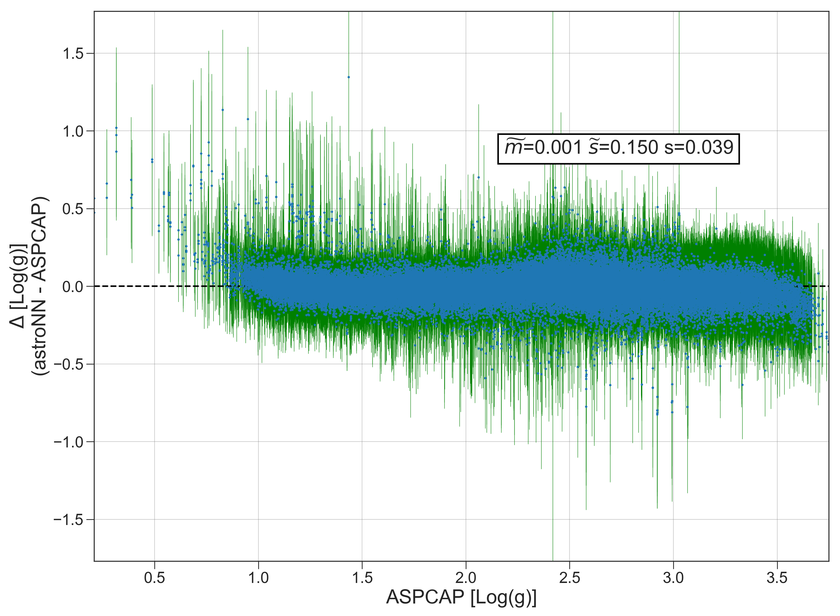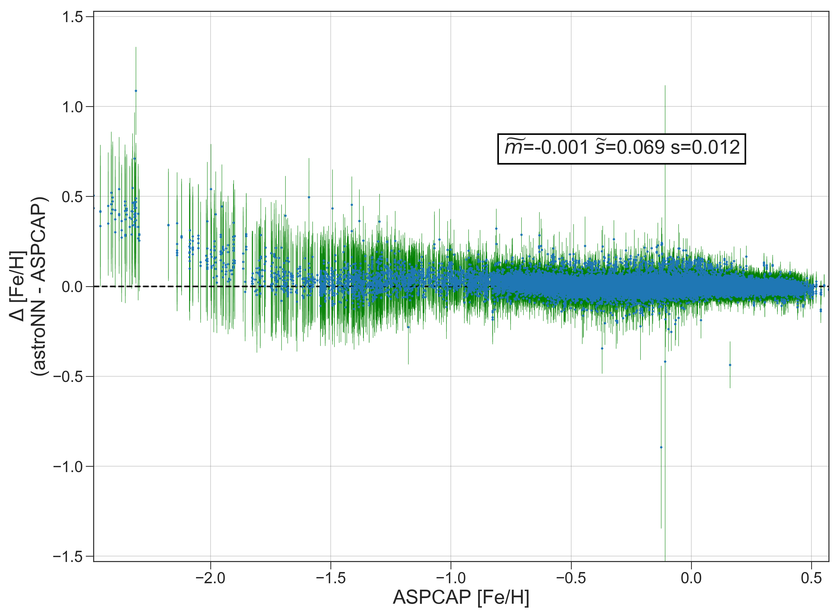## Example Plots using jacobian¶